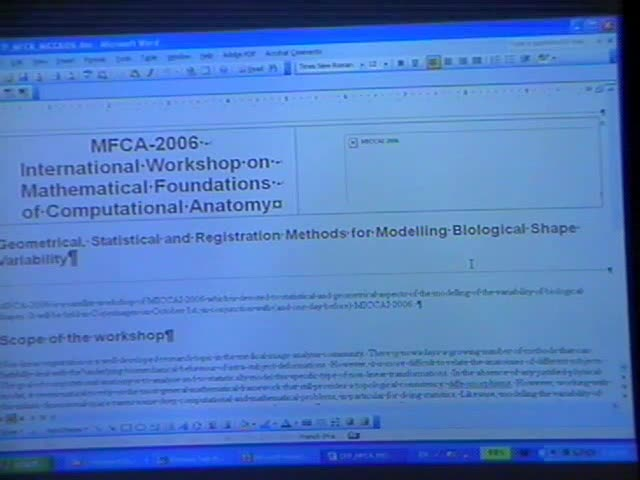### Videos

#### Statistics of Shape: Simple Statistics on Interesting Spaces

##### Presenter
April 5, 2006
Keywords:
• Statistical Models
MSC:
• 60K35##### Abstract
A primary goal of Computational Anatomy is the statistical analysis of anatomical variability. A natural question that arises is how dose one define the image of an "Average Anatomy" given a collection of anatomical images. Such an average image must represent the intrinsic geometric anatomical variability present. Large Deformation Diffeomorphic transformations have been shown to accommodate the geometric variability but performing statistics of Diffeomorphic transformations remains a challenge. Standard techniques for computing statistical descriptions such as mean and principal component analysis only work for data lying in a Euclidean vector space. In this talk, using the Riemannian metric theory the ideas of mean and covariance estimation will be extended to non-linear curved spaces, in particular for finite dimensional Lie-Groups and the space of Diffeomorphisms transformations. The covariance estimation problem on Riemannian manifolds is posed as a metric estimation problem. Algorithms for estimating the "Average Anatomical" image as well as for estimating the second order geometrical variability will be presented.